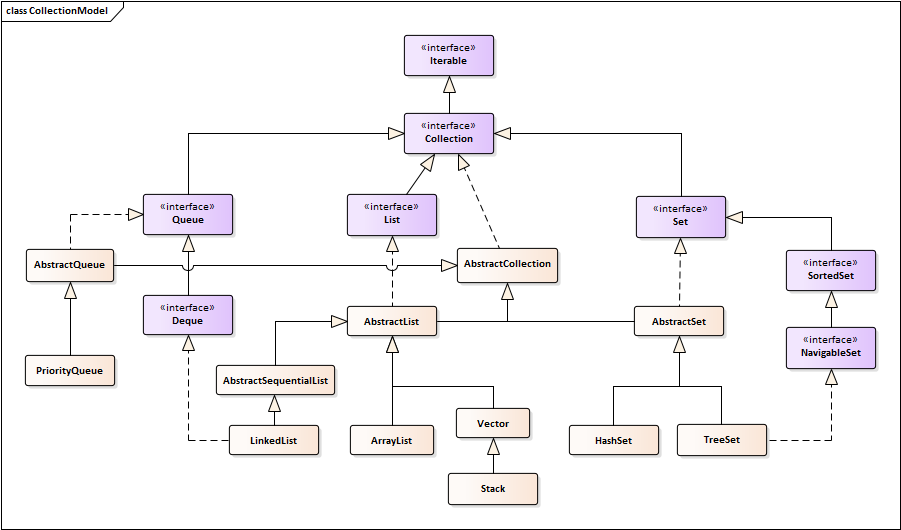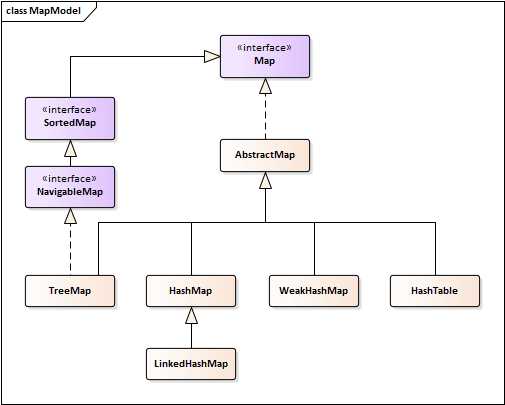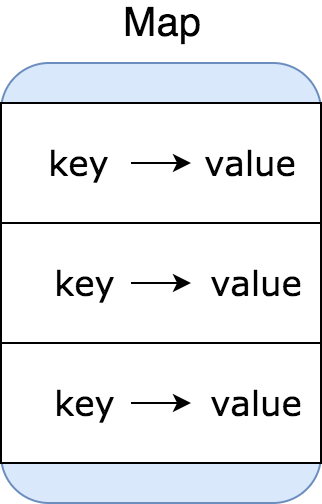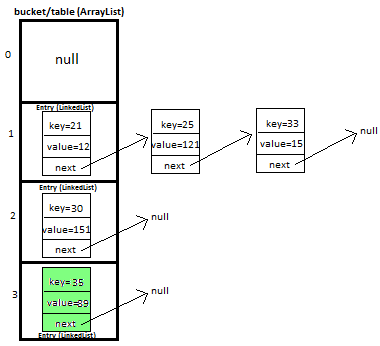# Java Collection II - Map in JavaCollection总体框架图

Java集合主要有List集合，Set集合，Map映射以及工具类（Arrays，Collections，Iterator），上图是继承Collection接口的类。Map是唯一一个不继承或实现Collection的集合，框架图如下。Map总体框架图

# 什么是Map

Map是专为快速查找而设计的数据结构。数据在Map中以键值对的方式存储，每组键值对称为一个entry，并且不允许插入重复的键。Map的实现包含TreeMap、HashMap、WeakHashMap、HashTable和LinkedHashMap，最常用的是HashMap，以下大多数的代码使用HashMap作为例子。JDK1.8中的HashMap的哈希桶采用链表和红黑树互相转化的实现，也就是当链表大小超过一定阈值的时候，会自动转化为一颗红黑树，默认阈值为`TREEIFY_THRESHOLD=8`## 创建Map

Map的键值类型只能是引用类型，不能使用基本数据类型。例如我们可以创建一个Integer类型的key和String类型的value的HashMap。

``````Map<Integer, String> map = new HashMap<>();
``````

``````Map<Integer, String> map = new HashMap<>(anotherMap);
``````

HashMap允许插入一个`null`键和多个`null`值，并且不保存元素的顺序。

## 基本操作

• `put`插入一个键值对，如果map存在一个相同的key，则返回更新前的值；否则返回`null`
• `putAll`与构造函数`HashMap(Map<? extends K, ? extends V> m)`相似，均调用`putMapEntries`，向当前map中插入m的所有条目；
• `putIfAbsent`则会判断是否存在相同的key，若存在则不更新对应的value；否则插入新条目；

• `get`返回对应的value或者`null`
• `getOrDefault`函数在key不存在的情况下返回一个默认值；

• keySet()，返回map中的所有键
• values()，返回map中的所有值
• entrySet()，返回所有<key,value>条目

# Map的遍历

map的遍历方式有很多种，这里我们列举几种常用的方式。

## foreach与`entrySet`

entrySet视图经常用来遍历一个map，这里要注意map不能为`null`，否则会报`NullPointerException`。这种方法效率高。

``````for(Map.Entry entry : map.entrySet()) {
System.out.println(entry.getKey() + ": " + entry.getValue());
}
``````

## Lambda表达式

Java 8中引入了Lambda表达式，一种更紧凑的遍历写法可以是下面这样的：

``````map.forEach((k,v) -> System.out.println(k + ": " + v));
``````

## Iterator迭代器

``````Iterator<Map.Entry<Integer, String>> it = map.entrySet().iterator();
while(it.hasNext()) {
Map.Entry<Integer, String> entry = it.next();
System.out.println(entry.getKey() + ": " + entry.getValue());
}
``````

# Map的排序

HashMap并不会为entries保留一个顺序，若要使得所有条目按照key排序，可使用TreeMap。TreeMap是基于红黑树实现的映射，它可以根据key的自然排序为所有entries保留顺序，也可以在构造的时候提供默认的Comparator。`get`/`put`/`containsKey`的时间复杂度为\$\log{n}\$。与HashMap一样，TreeMap也是线程不安全的，若要在多线程环境使用，需要在外部做好线程同步操作。

``````Map<Integer, String> map = new TreeMap<>();
map.put(1, "A");
map.put(2, "B");
map.put(3, "C");

map.forEach((k, v) -> System.out.println(k + ": " + v));
``````

``````1: A
2: B
3: C
``````

``````private static <K extends Comparable, V extends Comparable> Map<K, V> sortMapByValue(Map<K, V> map) {
List<Map.Entry<K, V>> entries = new LinkedList<>(map.entrySet());
Collections.sort(entries, new Comparator<Map.Entry<K, V>>() {
@Override
public int compare(Map.Entry<K, V> o1, Map.Entry<K, V> o2) {
return o1.getValue().compareTo(o2.getValue());
}
});
Map<K, V> sortedMap = new LinkedHashMap<>();

for(Map.Entry<K, V> entry : entries) {
sortedMap.put(entry.getKey(), entry.getValue());
}

return sortedMap;
}
``````

# 浅拷贝与深拷贝

``````public class Student {
private String name;

// constructor, getters and setters
}
``````

## Shallow copy

``````Set<Entry<String, Student>> entries = originalMap.entrySet();
HashMap<String, Student> shallowCopy = (HashMap<String, Student>) entries.stream()
.collect(Collectors.toMap(Map.Entry::getKey, Map.Entry::getValue));
``````

## Deep copy

``````public class Student implement Serializable {
private String name;

// constructor, getters and setters
}
HashMap<String, Student> deepCopy = SerializationUtils.clone(originalMap);
``````

# 算法题

## 同构字符串

Input: `s="egg"``t="add"`
Output: true

``````class Solution {
public boolean isIsomorphic(String s, String t) {
if(s == null || s.isEmpty()) return true;
int p = 0;
HashMap<Character, Character> map = new HashMap<>();
while(p < s.length()) {
if(map.containsKey(s.charAt(p))) {
if(!map.get(s.charAt(p)).equals(t.charAt(p))) return false;
}
else {
if(!map.containsValue(t.charAt(p))) {
map.put(s.charAt(p), t.charAt(p));
}
else return false;
}
++p;
}
return true;
}
}
``````

## 最长协调子序列

LeetCode简单难度594题，要求在给定数组中找到最长的协调子序列。

Input: [1,3,2,2,5,2,3,7]
Output: 5
Note: 最长协调子序列为[3,2,2,2,3]

``````class Solution {
public int findLHS(int[] nums) {
Arrays.sort(nums);
int len = 0;
int left = 0, right = 1;
while(right < nums.length) {
while(left < nums.length && nums[right] - nums[left] > 1) ++left;
if(nums[right] - nums[left] == 1) len = Math.max(right-left+1, len);
++right;
}
return len;
}
}
``````

``````class Solution {
public int findLHS(int[] nums) {
Map<Integer, Integer> map = new HashMap<>();
for(int n : nums) {
map.put(n, map.getOrDefault(n, 0)+1);
}
int res = 0;
for(int n : nums) {
if(map.containsKey(n+1)) {
res = Math.max(res, map.get(n)+map.get(n+1));
}
}
return res;
}
}
``````